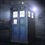# Help needed friends!

$x,y \in Z^{+}\\ (x+y)^2+3x+y=1994\\ y=?$5 years, 9 months ago

This discussion board is a place to discuss our Daily Challenges and the math and science related to those challenges. Explanations are more than just a solution — they should explain the steps and thinking strategies that you used to obtain the solution. Comments should further the discussion of math and science.

When posting on Brilliant:

• Use the emojis to react to an explanation, whether you're congratulating a job well done , or just really confused .
• Ask specific questions about the challenge or the steps in somebody's explanation. Well-posed questions can add a lot to the discussion, but posting "I don't understand!" doesn't help anyone.
• Try to contribute something new to the discussion, whether it is an extension, generalization or other idea related to the challenge.

MarkdownAppears as
*italics* or _italics_ italics
**bold** or __bold__ bold
- bulleted- list
• bulleted
• list
1. numbered2. list
1. numbered
2. list
Note: you must add a full line of space before and after lists for them to show up correctly
paragraph 1paragraph 2

paragraph 1

paragraph 2

[example link](https://brilliant.org)example link
> This is a quote
This is a quote
    # I indented these lines
# 4 spaces, and now they show
# up as a code block.

print "hello world"
# I indented these lines
# 4 spaces, and now they show
# up as a code block.

print "hello world"
MathAppears as
Remember to wrap math in $$ ... $$ or $ ... $ to ensure proper formatting.
2 \times 3 $2 \times 3$
2^{34} $2^{34}$
a_{i-1} $a_{i-1}$
\frac{2}{3} $\frac{2}{3}$
\sqrt{2} $\sqrt{2}$
\sum_{i=1}^3 $\sum_{i=1}^3$
\sin \theta $\sin \theta$
\boxed{123} $\boxed{123}$

Sort by:

To make this problem much simpler, let us make a substitution $x = \dfrac{u+v}{2}$ and $y=\dfrac{u-v}{2}$.

So, the given equation converts into

$u^2 + 2u + \left( v- 1994 \right) = 0$

Step-1: Let us first find the lower bound and upper bound of $v$. It is clear that $u = x+y$ and $v = x-y$. So, $u > v$ using this fact we get

\begin{aligned} 1994 = u^2 + 2u +v > v^2 + 3v \\ v^2 + 3v - 1994 < 0 \end{aligned}

Since $x$ and $y$ are integers and so $v$ is an integer. Then from the above inequality, we get $v \in \Big[ -92, 86 \Big]$.

Step-2: Since from the equation $u^2 + 2u + \left( v- 1994 \right) = 0$. We get, $u= -1 \pm \sqrt{1995-v}$. But since $x>0$ and $y>0$ it follows that $u>0$. So, $u= -1 + \sqrt{1995-v}$.

Now for positive integral value of $u$, $1995-v$ should be a perfect square. Now from the step-1 $1995-v \in \Big[ 1909, 2087 \Big]$. There are only two values of $1995 -v$, they are $2025$ and $1936$.

They yield the following pairs of $\left(u,v \right)$. They are $\{ \left(43,59 \right) , \left(44, -30 \right) \}$. But since $u>v$, we get only pair of $\left( u,v\right)$ i.e. $\left( 44, -30 \right)$ which yields $x = 7$ and $y= 37$.

So, therefore only one solution of $\left( x , y \right)$ exists i.e. $\left( 7 , 37 \right)$.

- 5 years, 9 months ago

Thanx for the solution!An awesome one!

- 5 years, 9 months ago

- 5 years, 9 months ago

X=7 and Y=37.

- 5 years, 9 months ago

What have you tried? Where are you stuck?

Staff - 5 years, 9 months ago

I tried a bit of modular arithmetic and tried factorising the expression but nothing worked.A hint sir?

- 5 years, 9 months ago

Well, for one, since $x, y > 0$, we see that $x, y < 2000$ and so we have finitely many possibilities to try.

Staff - 5 years, 9 months ago

$(x+y)^2 + (x + y) = 1994 - 2x$$\rightarrow (x + y )(x + y + 1) = 1994 - 2x$ Now as 1994 = 44.45 + 14, x = 7 and x + y = 44 is one and only valid solution.

- 5 years, 9 months ago

Well,thank you sir,a nice solution!

- 5 years, 9 months ago

I'm getting $x=7,y=37$

- 5 years, 9 months ago